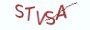Java基础教程

# 8.2.1 方法的重写

```class Dog{
public void catch_(){
System.out.println("狗拿耗子，多管闲事");
}
}

class Corgi extends Dog{
// 重写继承而来的catch_方法，柯基犬已经厌恶了学猫抓耗子
public void catch_(){
System.out.println("勾搭萝莉，成为舔狗");
}
}

public class HelloJava{
public  static void main(String[] args) {
Dog busyDog = new Dog();
busyDog.catch_();
Dog corgi = new Corgi();
corgi.catch_();
}

}```

(1) 子类方法的函数名，参数列表，返回值类型须与父类方法一致。

(2) 子类方法的访问权限须大于等于父类方法的访问权限

(3) 只能重写继承而来的对象方法，所以类方法和private方法均无法被重写

```class Dog{
public void catch_(){
System.out.println("狗拿耗子，多管闲事");
}
}

class Corgi extends Dog{
// 重写继承而来的catch_方法，柯基犬已经厌恶了狗拿耗子
@Override
public void catch_(){
System.out.println("勾搭萝莉，成为舔狗");
}
}

public class HelloJava{
public  static void main(String[] args) {
Dog busyDog = new Dog();
busyDog.catch_();
Dog corgi = new Corgi();
corgi.catch_();
}

}```

# 8.2.2 方法的重载

```class Dog{
public void catch_(){
System.out.println("狗拿耗子，多管闲事");
}
}

class Corgi extends Dog{
// 重写继承而来的catch_方法，柯基犬已经厌恶了狗拿耗子
@Override
public void catch_(){
System.out.println("勾搭萝莉，成为舔狗");
}

public void catch_(boolean isAMouse){
if ( isAMouse ) {
System.out.println("拒绝狗拿耗子");
} else {
System.out.println("不再狗拿耗子，做一只懒萌的柯基犬");
}
}
}

public class HelloJava{
public  static void main(String[] args) {
Corgi corgi = new Corgi();
corgi.catch_();
corgi.catch_(false);
}
}```

```class Statistics{
public static int sum(int a, int b){

return a+b;
}

public static double sum(int a, double b){
return a+b;
}

public static double sum(double a, double b){
return a+b;
}

}```

Java在匹配重载方法时，优先匹配参数个数一致的函数，如果参数个数不一致，Java会立即抛出错误异常。如果参数个数一致，再寻找参数类型完全匹配的方法。如果参数类型不一致，则继续寻找参数能隐式转换为目标参数类型的重载方法。这里的隐式转换是指小范围的数据类型向上提升为范围更大的数据类型，匹配的策略采取就近原则进行匹配。在以上三个重载方法中，如果按以下方式来调用sum方法：

```class Statistics{
public static int sum(int a, int b){ return a+b; }

public static double sum(int a, double b){
return a+b;
}

public static double sum(double a, double b){
return a+b;
}

}

public class HelloJava{
public  static void main(String[] args) {
int a = 1;
int b = 1;
int value = Statistics.sum(a, b);
System.out.println(value);
}

}```

```class Statistics{
public static int sum(int a, int b){ return a+b; }

public static double sum(int a, double b){
return a+b;
}

public static double sum(double a, double b){
return a+b;
}

}

public class HelloJava{
public  static void main(String[] args) {
char a = 1;
char b = 1;
int value = Statistics.sum(a, b);
System.out.println(value);
}

}```

```class Statistics{
/*public static int sum(int a, int b){ return a+b; } */

public static double sum(int a, double b){ return a+b; }

public static double sum(double a, double b){
return a+b;
}

}

public class HelloJava{
public  static void main(String[] args) {
char a = 1;
char b = 1;
double value = Statistics.sum(a, b);
System.out.println(value);
}

}```

# 8.2.4 课后习题

(1) 何为方法的重写？为什么要对方法进行重写？

(2) 写一个方法重写的代码实例，以回答你为什么要对方法进行重写。

(3) 什么是方法的重载？可以对重写后的方法进行重载吗？

(4) 简述重写与重载的区别。

(5) 定义一个编程语言类，要求至少设计三个重载方法。

(6) 请用一句话概括修改和扩展之间的联系。

# 8.2.5 高薪就业班

`(1) Python后端工程师高薪就业班，月薪10K-15K，免费领取课程大纲(2) Python爬虫工程师高薪就业班，年薪十五万，免费领取课程大纲(3) Java后端开发工程师高薪就业班，月薪10K-20K, 免费领取课程大纲(4) Python大数据工程师就业班，月薪12K-25K,免费领取课程大纲`## 注册账号